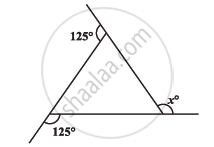Share

# Find X In the Following Figures - CBSE Class 8 - Mathematics

ConceptSum of the Measures of the Exterior Angles of a Polygon

#### Question

Find x in the following figures#### Solution

We know that the sum of all exterior angles of any polygon is 360º.

125° + 125° + x = 360°

250° + x = 360°

x = 110°

Is there an error in this question or solution?

#### APPEARS IN

NCERT Solution for Mathematics Textbook for Class 8 (2018 to Current)